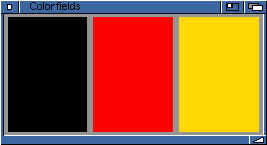## 3.4 Group objects

As mentioned above, a programmer specifies a window's design by grouping objects either horizontally or vertically. As a little example, let's have a look at a simple file requester window:This window consists of two listview objects, two string gadgets and two buttons. To tell MUI how these objects shall be placed, you need to define groups around them. Here, the window consists of a vertical group that contains a horizontal group with both lists as first child, the path gadget as second child, the file gadget as third child and again a horizontal group with both buttons as fourth child.

In an XML file, the GUI definition of this window could then look like this:

 ```
```

This XML definition is completely enough to define the contents of our window, all necessary sizes and positions are automatically calculated by the MUI system.

To understand how these calculations work, it's important to know that all basic objects (e.g. strings, buttons, lists) have a fixed minimum and a maximum size. Group objects calculate their minimum and maximum sizes from their children, depending whether they are horizontal or vertical:

Horizontal groups:

• – The minimum width of a horizontal group is the sum of all minimum widths of its children.

• – The maximum width of a horizontal group is the sum of all maximum widths of its children.

• – The minimum height of a horizontal group is the biggest minimum height of its children.

• – The maximum height of a horizontal group is the smallest maximum height of its children.

Vertical groups:

• – The minimum height of a vertical group is the sum of all minimum heights of its children.

• – The maximum height of a vertical group is the sum of all maximum heights of its children.

• – The minimum width of a vertical group is the biggest minimum width of its children.

• – The maximum width of a vertical group is the smallest maximum width of its children.

Maybe this algorithm sounds a little complicated, but in fact it is really straight forward and ensures that objects will neither get smaller as their minimum nor bigger as their maximum size.

Before a window is opened, it asks its root object (usually a group object) to calculate minimum and maximum sizes. These sizes are used as the window's bounding dimensions, the smallest possible window size will result in all objects being display in their minimum size.

Once minimum and maximum sizes are calculated, layout process starts. The root object is told to place itself in the rectangle defined by the current window size. This window size is either specified by the programmer or results from a window resize operation by the user. When an object is told to layout itself, it simply sets its position and dimensions to the given rectangle. In case of a group object, a more or less complicated algorithm distributes all available space between its children and tells them to layout too.

This "more or less complicated algorithm" is responsible for the object arrangement. Depending on some attributes of the group object (horizontal or vertical, ...) and on some attributes of the children (minimum and maximum dimensions, ...), space is distributed and children are placed.

A little example makes things more clear. Let's see what happens in a window that contains nothing but three horizontally grouped colorfield objects:Colorfield objects have a minimum width and height of one pixel and no maximum width and height. Since we have a horizontal group, the minmax calculation explained above yields to a minimum width of three pixels and a minimum height of one pixel for the window's root object (the horizontal group containing the colorfields). Maximum dimensions of the group are unlimited. Using these results, MUI is able to calculate the windows bounding dimensions by adding some spacing values and window border thicknesses.

Once min and max dimensions are calculated, the window can be opened with a programmer or user specified size. This size is the starting point for the following layout calculations. For our little example, let's imagine that the current window size is 100 pixels wide and 50 pixels high.

MUI subtracts the window borders and some window inner spacing and tells the root object to layout itself into the rectangle left=5, top=20, width=90, height=74. Since our root object is a horizontal group in this case, it knows that each colorfield can get the full height of 74 pixels and that the available width of 90 pixels needs to be shared by all three fields. Thus, the resulting fields will all get a width of 90/3=30 pixels.

That's the basic way MUI's layout system works. There are a lot more possibilities to influence layout, you can e.g. assign different weights to objects, define some inter object spacing or even make two-dimensional groups. These sophisticated layout issues are discussed in the documentation of group class.

Show TOC